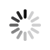#### Welcome!

×Forgot password?
Go back to Challenges list

Challenge

## Basic decryption

The alphabet used is: "j K a v P Z d i p M t q m 6 T L g F 7 G R l X A o 8 b r s 2 u 3 D x H z n B V w E S 0 y I J 1 f 9 N O 4 Q k h e U C W c Y 5".
This basic decryption changes every char to the next char on the given alphabet (the number between the hashes it's the number of jumps to the next character).
For example:
"#2#yXQH" is encrypted with 2 jumps in every character, that means:
y -> J
X -> o
Q -> h
H -> n
"John"
If the character is "5" and the number between the hashes is "1", "5" changes to "j"
If the character is "Y" and the number between the hashes is "4", "Y" changes to "a"

"##"

""

###### Sample Input
`#12#eyTLQ`

`Zebra`

`512M`